The perimeter is just the distance it would take if you were to walk the outside edges of a given shape. So, for the following triangle: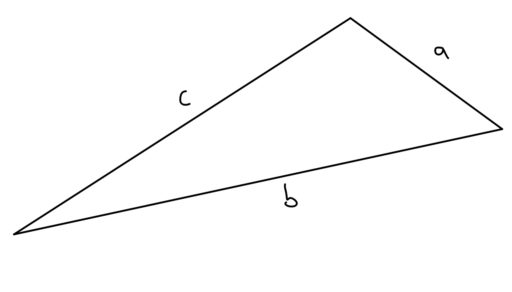the perimeter is justFinding the perimeter of a square or a rectangle is similarly straightforward:

Perimeter of a Square or Rectangle: In the following diagrams, the perimeter of the square isand the perimeter of the rectangle is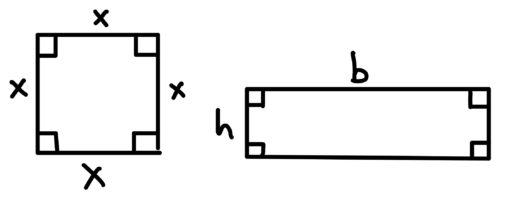Perimeter of a Rhombus: Since a rhombus is defined by having four sides of equal length, the perimeter of the below rhombus is just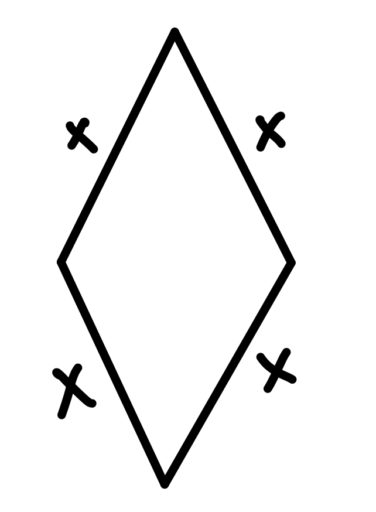Perimeter of a Trapezoid:

The perimeter of a trapezoid is a little trickier, but recall that we can break a trapezoid up into two right triangles and a rectangle: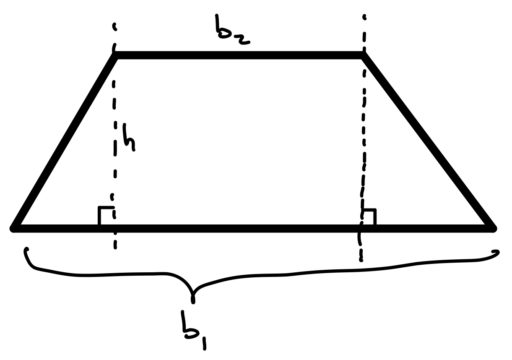And then, if we have the bases of the right triangles, we can use the Pythagorean Theorem to find the length of the diagonal bits: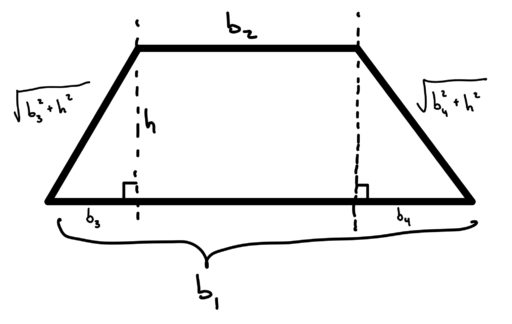Thus, we get that the perimeter is:Perimeter of a Parallelogram
Remember that the parallel sides of a parallelogram have the same length. Thus, for the below parallelogram: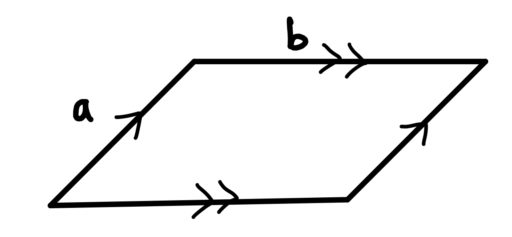the perimeter is just.

include some simpler problems here for finding the perimeter of when they give you basically all the right information

Sometimes, the perimeter figures in a word problem. So, for example, you might have:

Example 1

A farmer has a square field whose perimeter is twice its area. What is the area of the field?

Practice Problems

1. The outer rectangle below isunits apart from the smaller rectangle on the top and bottom sides, andunits apart on the left and right sides. The outer rectangle has a base of 8 units and a height of 12 units. What is the perimeter of the inner rectangle?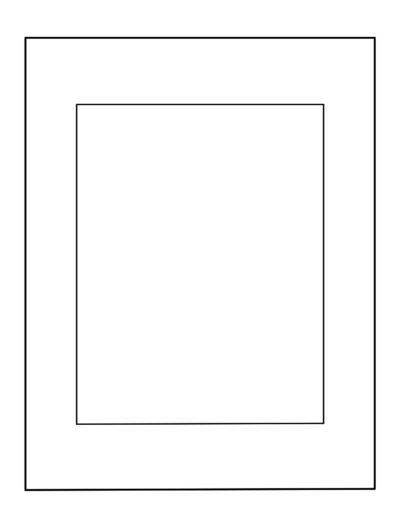2. The perimeter of a regular-sided shape isFind the length of a side.

3. The length of a side of a regular hexagon isWhat is the perimeter?